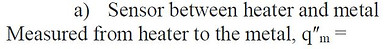###### Workshop #6 - Introduction to Heat Sinks and Energy Balances

Review Energy Balances

This workshop uses the heater to emphasize thermal resistance and the energy balance. Specifically, where does the thermal energy from the heater go and how does it get there? This is an important problem in many thermal systems. To make it simple, it will be assumed to be one-dimensional. On one side of the heater will be a low conductivity material and on the other will be a high conductivity material, both starting at the same temperature. When the heater is turned on, heat flux will go to each of the materials. The heat flux sensor can be used to measure how much goes to each material.

The energy balance can be used to show that the total power from the heater P has to go to the combination of the two materials. Draw the overall energy balance on the figure and write the algebraic energy balance here in terms of the heat transfer to the metal qm and the carpet qc.

The relative thermal resistances will dictate the relative size of qm and qc (how much energy goes to each material). Because there is only one heat flux sensor, you will need to use it twice. First to measure what goes to the metal and then what goes to the carpet.

1. Place the heater on a piece of carpet or other good thermal insulator and place the metal piece over top. Slide the heat flux sensor between the metal and the heater. Start the data acquisition to establish the steady-state condition then turn on the heater. Allow the heater to run for about one minute to clearly see the response of the temperature and heat flux. Next move the heat flux sensor between the heater and the carpet. Repeat the data acquisition for this case. Plot the heat flux and temperature responses for both cases. Add these plots to the this workshop sheet.

2. Draw the system for each case and clearly label the four main components: carpet, heater, sensor, and metal. Label the direction of the heat fluxes in each case – from the heater to the metal piece and the carpet.

3. Why are the heat fluxes so different in value?

4. Which material provides the better heat sink?

5. Why is one heat flux positive and one heat flux negative with the same sensor?

6. What is the total power flux provided by the heater? P/A =
.

7. What is the fraction of the power that goes to the metal, qʺm / (P/A) =

8. What is the temperature response for the two cases?

9. Why is the temperature response for the two cases so different than the heat flux?###### Workshop #6 - Heat Sinks and Energy Balances Results